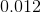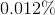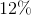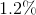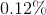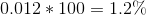# Algebra 1 : How to find percentage equivalent to a decimal

## Example Questions

### Example Question #11 : How To Find Percentage Equivalent To A Decimal

Find the percentage equivalent of the following decimal: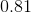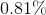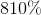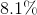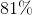Explanation:

In order to find the percentage equivalent for a decimal, all we have to do is multiply our decimal by 100. We can also move the decimal point to the right two spaces.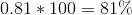### Example Question #12 : How To Find Percentage Equivalent To A Decimal

Find the percentage equivalent for the following decimal: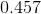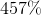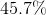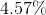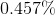Explanation:

In order to find the percentage equivalent for a decimal, all we have to do is multiply our decimal by 100. We can also move the decimal point to the right two spaces.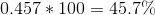### Example Question #13 : How To Find Percentage Equivalent To A Decimal

Find the percentage equivalent for the following decimal: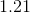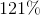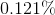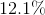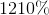Explanation:

In order to find the percentage equivalent for a decimal, all we have to do is multiply our decimal by 100. We can also move the decimal point to the right two spaces.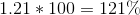### Example Question #14 : How To Find Percentage Equivalent To A Decimal

Find the percentage equivalent of the following decimal: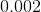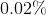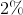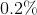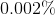Explanation:

In order to find the percentage equivalent for a decimal, all we have to do is multiply our decimal by 100. We can also move the decimal point to the right two spaces.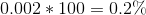### Example Question #15 : How To Find Percentage Equivalent To A Decimal

Find the percentage equivalent of the following decimal: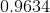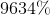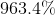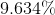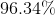Explanation:

In order to find the percentage equivalent for a decimal, all we have to do is multiply our decimal by 100. We can also move the decimal point to the right two spaces.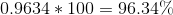### Example Question #16 : How To Find Percentage Equivalent To A Decimal

Find the percentage equivalent of the following decimal: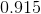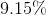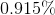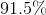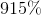Explanation:

In order to find the percentage equivalent for a decimal, all we have to do is multiply our decimal by 100. We can also move the decimal point to the right two spaces.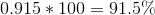### Example Question #17 : How To Find Percentage Equivalent To A Decimal

Find the percentage equivalent of the following decimal: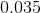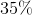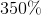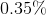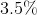Explanation:

In order to find the percentage equivalent for a decimal, all we have to do is multiply our decimal by 100. We can also move the decimal point to the right two spaces.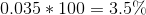### Example Question #18 : How To Find Percentage Equivalent To A Decimal

Find the percentage equivalent of the following decimal: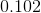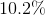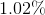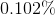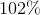Explanation:

In order to find the percentage equivalent for a decimal, all we have to do is multiply our decimal by 100. We can also move the decimal point to the right two spaces.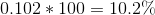### Example Question #19 : How To Find Percentage Equivalent To A Decimal

Find the percentage equivalent for the following decimal: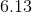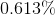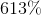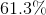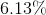Explanation:

In order to find the percentage equivalent for a decimal, all we have to do is multiply our decimal by 100. We can also move the decimal point to the right two spaces.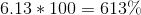### Example Question #20 : How To Find Percentage Equivalent To A Decimal

Find the percentage equivalent of the following decimal: